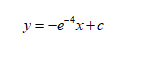# Find an equation of the tangent line to the graph of y = e−x^2 at the point (4, 1/e16).

Question
121 views

Find an equation of the tangent line to the graph of

y = e−x^2 at the point (4, 1/e16).
check_circle

Step 1

Slope of tangent line is equal to value of the first derivative of y at given point.

Step 2

So the equation of the line is of the formStep 3

Substitute the given point to ...

### Want to see the full answer?

See Solution

#### Want to see this answer and more?

Solutions are written by subject experts who are available 24/7. Questions are typically answered within 1 hour.*

See Solution
*Response times may vary by subject and question.
Tagged in

### Functions